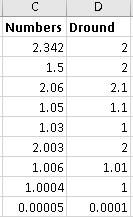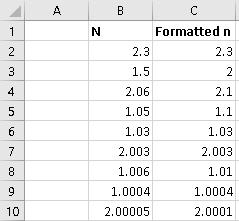# Automatically Formatting for Decimal Placesby Allen Wyatt
(last updated January 14, 2021)

John has a data entry worksheet that allows users to enter information. He would like to have a cell be automatically formatted to display exactly the number of decimal places that a user types. For example, if the user types "12.345" then he would like the cell to be automatically formatted to display 3 decimal places. John knows he could use the General format for a cell (which does this nicely), but that approach doesn't work if the user enters a value that ends in 0, such as "12.34500", which he would want formatted (automatically) to display 5 decimal places.

If you are thinking that you could use a custom format to address the need, that won't work. With ever custom format we could come up with, Excel drops any trailing zeroes from what it displays. (Or, conversely, if the custom format includes "0" as a placeholder, it adds zeroes at the end of the entry.)

The easiest way to handle this, quite honestly, is to simply format the cells as Text before you start entering information. In that way, Excel will simply accept what is entered—including any trailing zeroes—and stuff it into the cell. You can, further, right-align the contents of the cells so that they at least look a bit more like numeric values.

The drawback to this is that you've got to be careful in using the values in formulas. The safest way is to simply surround any reference to the cell within the VALUE function, in this manner:

```=VALUE(A1) * 1.375
```

Another approach is to create a macro that checks what is entered into a range of cells. Start by formatting the cells as Text, and then create a named range (DataEntry) from those cells. You can then add the following code to the code sheet for the worksheet you are using:

```Private Sub Worksheet_Change(ByVal Target As Range)
Dim c As Range
Dim sEntry As String
Dim dEntryNumber As Double
Dim arr

If Not Intersect(Target, Range("DataEntry")) Is Nothing Then
Application.EnableEvents = False
For Each c In Target.Cells
If IsEmpty(c) Then
c.NumberFormat = "@"    ' Reset to Text format
Else
If IsNumeric(c) Then
If Len(c.Value) = 0 Then
c.NumberFormat = "@"    ' Reset to Text format
Else
sEntry = c.Value
dEntryNumber = CDbl(sEntry)

arr = Split(sEntry, ".")
If UBound(arr) = 1 Then
' Change NumberFormat in accordance with
' the number of digits after the decimal point
c.NumberFormat = "0." & String(Len(arr(1)), "0")
c.Value = dEntryNumber
End If
End If
End If
End If
Next c
Application.EnableEvents = True
End If
End Sub
```

The macro is triggered everytime something changes in the worksheet. It then checks to see if that change occurred in one of the cells in the DataEntry range. If so, then it examines what is entered in the cell (which Excel treats as text, since that's how the cell was formatted) and determines if it is a number and further how many digits there are to the right of the decimal place. It then formats the cell to have that many decimal places showing and stuffs the numeric value back into the cell.

The only condition where this approach won't work is if you place a value into a cell in the DataEntry range (which converts the cell to a numeric format) and then you enter a different numeric value in the same cell. The macro has no way of knowing, in that instance, if there are any trailing zeroes being entered. (Remember that trailing zeroes are only retained if the cell is formatted as Text. Since the cell is not, Excel lops off the trailing zeros and the macro works with that value as if it had been entered.)

Note:

If you would like to know how to use the macros described on this page (or on any other page on the ExcelTips sites), I've prepared a special page that includes helpful information. Click here to open that special page in a new browser tab.

ExcelTips is your source for cost-effective Microsoft Excel training. This tip (1963) applies to Microsoft Excel 2007, 2010, 2013, and 2016.

##### Author Bio

Allen Wyatt

With more than 50 non-fiction books and numerous magazine articles to his credit, Allen Wyatt is an internationally recognized author. He is president of Sharon Parq Associates, a computer and publishing services company. ...

##### MORE FROM ALLEN

Specifying a Location To Save Automatic Backup Files

When Word creates automatic backups of your documents, you may not like where Word stores them. This naturally leads to ...

Discover More

Splitting Information into Rows

Got too much information in a single cell? Here's how you can use a macro to pull apart that information and put it into ...

Discover More

Counting Cells Containing a Formula

Cells in a worksheet can contain different types of information, such as numbers, text, and formulas. If you want to ...

Discover MoreSave Time and Supercharge Excel! Automate virtually any routine task and save yourself hours, days, maybe even weeks. Then, learn how to make Excel do things you thought were simply impossible! Mastering advanced Excel macros has never been easier. Check out Excel 2010 VBA and Macros today!

##### More ExcelTips (ribbon)

Referencing External Cell Colors

If you want to reference cell colors external to your current workbook, there is no way to do it using Excel functions. ...

Discover More

Understanding Cell Indenting

Formatting a cell could, if you desire, also include the indentation of information within the cell. This tip examines ...

Discover More

Shortcut to Merge Cells

Need to merge a bunch of cells together on a regular basis? You'll love the two macros in this tip which can make short ...

Discover More
##### Subscribe

FREE SERVICE: Get tips like this every week in ExcelTips, a free productivity newsletter. Enter your address and click "Subscribe."

If you would like to add an image to your comment (not an avatar, but an image to help in making the point of your comment), include the characters [{fig}] in your comment text. You’ll be prompted to upload your image when you submit the comment. Maximum image size is 6Mpixels. Images larger than 600px wide or 1000px tall will be reduced. Up to three images may be included in a comment. All images are subject to review. Commenting privileges may be curtailed if inappropriate images are posted.

What is eight more than 6?

2020-01-20 19:00:38

Peter Atherton

GANESH
On further testing I realised that the UDF was rubbish and started again; this is wahat you get when working at bedtime. The following is correct.

Function DynamicRound(ByVal Value As Variant) As Double
Dim i As Integer, dec As Variant
Dim L As Integer, dSig, st As Integer
Dim dMid As Variant
dSig = False
st = Len(Value) - Application.Find(".", Value, 1)
dec = Right(Value, st)
For i = 1 To Len(dec)
dMid = mid(dec, i, 1) + 0
If dMid > 0 Then
dSig = True
Else
L = L + 1
End If
If dSig Then
DynamicRound = Application.Round(Value, L)
Exit For
End If
Next i
End Function

The capture shows typical values

(see Figure 1 below)Figure 1.

2020-01-18 15:00:03

Peter Atherton

GANESH

Here is a UDF for the rounding.

Function DynamicRound(ByVal value As Variant) As Double
Dim i As Integer, dec As Double
Dim x, L As Integer
'If you have smaller values, add to start of array
x = Array(0.00005, 0.0005, 0.005, 0.05, 0.5)
dec = value - Int(value)

For i = LBound(x) To UBound(x)
If i = UBound(x) Then
DynamicRound = Application.Round(value, 0)
Exit For
ElseIf dec >= x(i) And dec < x(i + 1) Then
L = Len(x(i + 1)) - 2
DynamicRound = Application.Round(value, L)
Exit For
End If
Next i
End Function

You can enter it as =DYNAMICROUND(B2) if the value is in B2
or you can enter a formula instead of a reference
=DynamicRound(9/7) -->(returns 1.3)

2020-01-17 20:53:37

Peter Atherton

GANESH KHATRI
Are the results below what you want? If so do you want them to change automatically like Allen's sub or do you want for format the values using the selection?

(see Figure 1 below)Figure 1.

2020-01-16 15:33:52

GANESH KHATRI

Hi there,
I am looking to format the decimal place of each cell based on values in cell. For example, if the number is > than 0.5, round off to zero decimal and if the number is in the range from 0.05<n<0.5 one decimal and if the number is range 0.005<n<0.05 two decimal and etc.

Let me know if we can do something about it.

2016-03-11 19:00:27

Peter Atherton

Mark

It is but you will lose the formatting with calculations. 10.45000 + 2 will result in 12.45 as only the significant figures are calculated.

To retain the format will need a text calc function, like this?

Function CalcTextNumb(ByVal ref As Range, _
Multiplier As Range, _
Operator As String) As String
Dim dp As Integer, dpx As Integer
Dim y As String, x As String, i As Integer

Select Case Operator
Case Is = "+"
y = ref + Multiplier
Case Is = "-"
y = ref - Multiplier
Case Is = "*"
y = ref * Multiplier
Case Is = "/"
y = ref / Multiplier

End Select

dp = (Len(ref) - 1) - Len(y) + 1
'
If Len(ref) = Len(y) Then
CalcTextNumb = y
Else: GoTo format
End If
format:
x = y
For i = 1 To dp
x = x & 0
Next
CalcTextNumb = x
End Function

2016-03-07 12:50:24

Mark Marikos

A simpler solution would be to enter the value as text (as described above) and then have a second non-entry field that calculates (converts) the entered field as a number value. then use the number value field for any calculations.

##### This Site

Got a version of Excel that uses the ribbon interface (Excel 2007 or later)? This site is for you! If you use an earlier version of Excel, visit our ExcelTips site focusing on the menu interface.